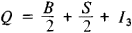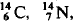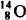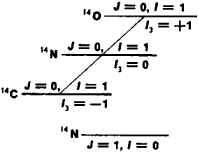# Isotopic Invariance

The following article is from The Great Soviet Encyclopedia (1979). It might be outdated or ideologically biased.

## Isotopic Invariance

a property of strong interactions of elementary particles. Particles that exist in nature and that have strong interactions (hadrons) may be divided into groups of “like” particles, each of which includes particles with approximately equal mass and identical internal characteristics (spin, baryon charge, and strangeness), with the exception of electric charge. Such groups are called isotopic multiplets. The strong interaction is found to be identical for all particles belonging to a given isotopic multiplet (that is, it does not depend on the electric charge); herein lies the symmetry of strong interactions, called isotopic invariance.

The simplest examples of particles that can be combined into a single isotopic multiplet are the proton (p) and the neutron (n). Experiments have shown that strong interaction between proton and proton, neutron and neutron, and proton and neutron is identical (if they are in the corresponding identical states); this was the starting point for the establishment of isotopic invariance. The proton and neutron are regarded as two different charged states of a single particle (the nucleon); they form an isotopic doublet. Other examples of isotopic multiplets are the pi-mesons (π+, π0, and π-) and sigma-hyperons (Σ+, Σ0, and Σ-), which form isotopic triplets.

The electric charge Q of a particle belonging to an isotopic multiplet is expressed by the Gell-Mann-Nishijima formula:Here B is the baryon charge, S is the strangeness (which is identical for all particles in a given isotopic multiplet), and the quantity I3 covers, at unit intervals, all values from some maximum value / (that is, integral or half-integral) to a minimum value equal to —I: h = I, I — …, — I. The total number of values that may be assumed by the quantity I3 (and by Q) for a given isotopic multiplet, and consequently the number of particles in an isotopic multiplet, is equal to 2I + 1. The quantity I, which defines the number of particles in an isotopic multiplet, is called the isotopic spin, and the quantity I3 is called the projection of the isotopic spin. These names are based on the formal mathematical analogy with the ordinary spin of particles, since according to quantum mechanics, for particles having a spin J the projection of the spin in an arbitrary direction in space may assume, at unit intervals, values ranging from +J to -J; that is, they may have 2J + 1 values.

Since nucleons exist in two charged states, for them (as for all other particles belonging to isotopic doublets) 2I + 1 = 2, that is, I = 1/2, and I3 may assume two values: +1/2 for a proton (corresponding to Q = +1, since nucleons have a baryon charge B = 1, and strangeness S = 0) and —1/2 for a neutron (Q = 0). The value I = 1 corresponds to an isotopic triplet of pions, and I3 is equal to + 1 for π +, 0 for π0, and — 1 for π-. Particles with I = 0 do not have isotopic “partners” and are isotopic singlets; Λ0 and Ω- hyperons are such particles.

Isotopic spin is thus an important characteristic of a hadron—the quantum number, which indicates how many isotopic partners a given particle has (or in how many charged states it may exist).

The existence, mass, and charge of new particles may be predicted on the basis of isotopic invariance if their isotopic partners are known. The existence of π0, Σ0 Ξ0 were predicted in this manner on the basis of the known π+, π- and also Σ+, Σ- and Ξ-.

Isotopic invariance also exists for compound systems of hadrons, in particular atomic nuclei. The isotopic spin of a complex system is composed of the isotopic spins of the particles constituting the system, and addition is performed according to the same rules that are used for ordinary spin. Thus, a system consisting of two particles with isotopic spin of 1/2 (such as a nucleon) and 1 (such as a pi-meson) may have isotopic spin I = 1 + 1/2 = 3/2, or I = 1 – 1/2 = 1/2.

In nuclei, isotopic invariance is manifested in the existence of energy levels with different quantum numbers for different isobars (that is, nuclei that contain an identical number of nucleons and differ in electric charge). The nucleiandare an example: the ground states of the nuclei 14C and 14O and the first excited state of 14N form an isotopic triplet, I = 1 (see Figure 1). All quantum numbers of these levels are identical, and the difference in their energies may be explained in terms of the difference in electrostatic energies because of the difference in the electric charges of these nuclei. (The ground state of 14N has isotopic spin I = 0, and therefore it has no analogues in the nuclei 14C and 14O.)

The law of conservation of total isotopic spin I in processes caused by strong interactions follows from isotopic invariance. This law leads to definite relations among the probabilities of processes for different particles belonging to identical isotopic multiplets, and also to the prohibition of certain reactions—for example, the reaction d + d → 4He + π cannot take place through strong interaction, since for d (a deuteron) and 4He, I = 0, but for the π0-meson I = 1. Many projects in high-energy charged-particle accelerators have been devoted to experimental verification of these predictions.Figure 1

Isotopic invariance exists only for strong interactions and is violated by electromagnetic interactions (which clearly depend on the electric charges of the particles—that is, on I3) whose “strength” is approximately 1 percent of that of strong interactions (by order of magnitude). The difference in electromagnetic interactions for different particles that belong to a given isotopic multiplet is responsible for the difference in their masses.

S. S. GERSHTEIN